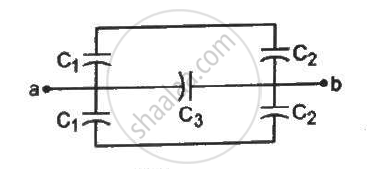Department of Pre-University Education, Karnataka course PUC Karnataka Science Class 12
Share

# Find the Equivalent Capacitance of the System Shown in Figure Between the Points a and B. - Physics

ConceptCapacitors and Capacitance

#### Question

Find the equivalent capacitance of the system shown in figure between the points a and b.#### Solution

Capacitors C1 and C2 above and below ab are connected in series and in parallel with C3.

Thus, the equivalent capacitor between a and b is given by

C_(eq) = (C_1C_2)/(C_1+C_2) + C_3 + (C_1C_2)/(C_1+C_2)

⇒ C_(eq) = C_3 + (2C_1C_2)/(C_1+C_2)

Is there an error in this question or solution?

#### Video TutorialsVIEW ALL 

Solution Find the Equivalent Capacitance of the System Shown in Figure Between the Points a and B. Concept: Capacitors and Capacitance.
S